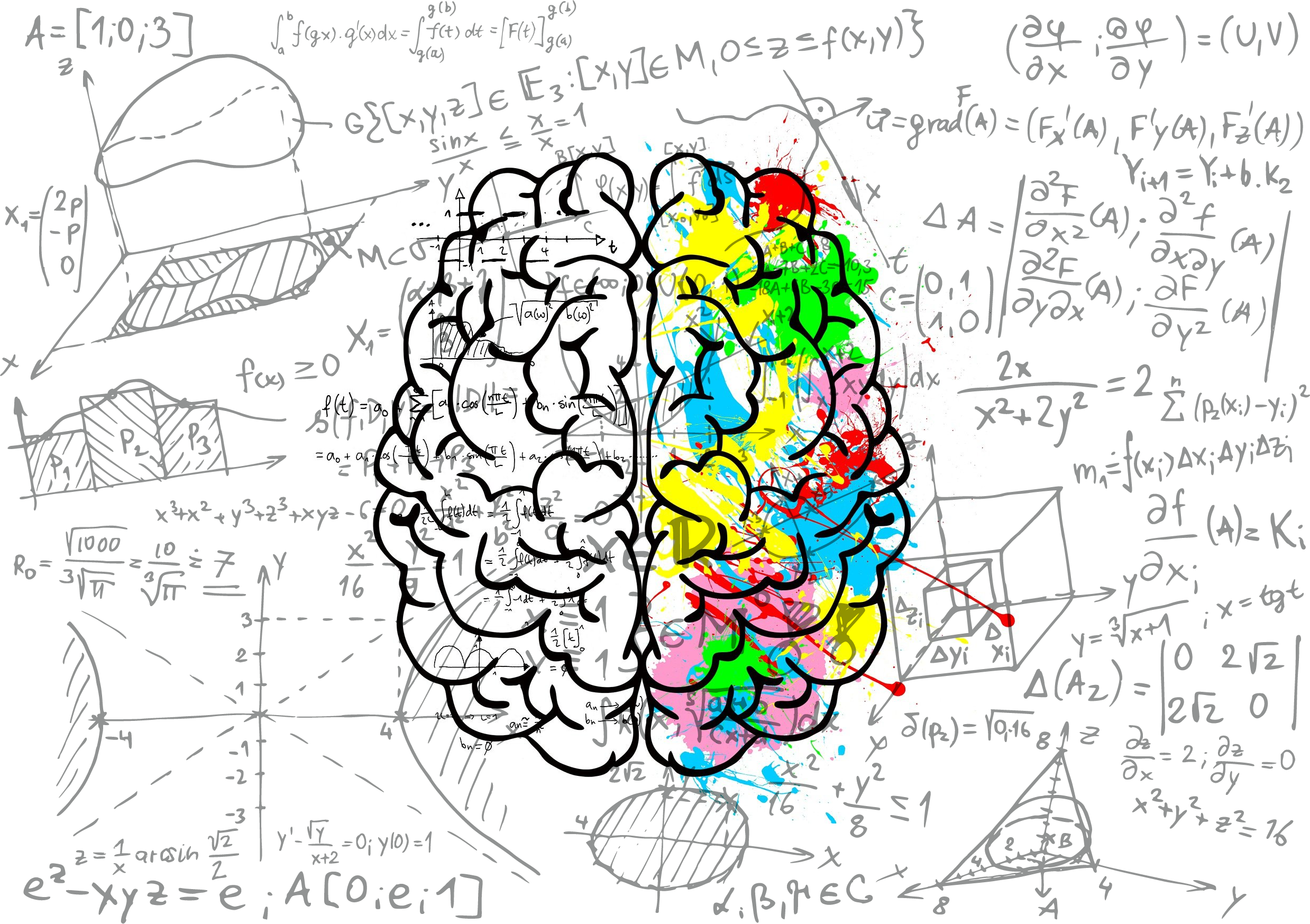April 23, 2019

# How do I identify the right Machine Learning model for my company?

Always confused? whether to go with shallow or deep learning models? This blog tries to answer that questionWritten by

### Tara McWhite

This blog outlines the differences between shallow and deep models from a practical perspective. We will review some of the key biases in machine learning, such as high bias/variance problems , data leakage and overfitting . Finally, we’ll apply these concepts to several examples for which you can try different approaches. Best of all, you don’t need to be an expert in mathematics or even coding to understand this topic; it’s intended for everyone who wants to make better use of machine learning technology.

For each example below, we compare shallow model training with a deep model (i.e., one that has many layers). We can’t cover all possible combinations of shallow and deep models, but this should give you a nice overview.

Shallow vs. Deep Algorithms

In general, by “shallow” we mean classification or regression algorithms that can be trained with only one hidden layer-for example: decision trees, logistic regression or neural networks with no convolutional layers (like some types of DNNs). By “deep,” we mean the same sets of algorithms augmented to include at least one convolutional layer (or two consecutive fully connected layers). We may also call such models “wide.” For example: ResNet-50.

We could use many different names for these classes of algorithm besides shallow and deep, including classical and modern , complex and simple, powerful and weak or others. But for this blog we will use shallow vs. deep since they correspond to two opposing viewpoints when discussing machine learning:

Shallow models are useful for problems with low bias (e.g., classification/regression problems) but high variance (e.g., overfitting). Deep models are useful for high bias problems like large datasets or sparse data but suffer from high variance.

Some dismiss the label of classical algorithms being shallow as misleading, arguing that many classical models require more parameters than you might initially think because they often rely on a random initialization . For example, relying on feature selection can reduce the number of parameters needed substantially by only choosing relevant features ; similarly, regularization techniques like L2-regularization or Dropout can reduce the need for more training examples. Lastly, Bayesian approaches are often seen as a way to decrease both bias and variance ; it does introduce some computational overhead but many consider it a worthwhile tradeoff.

The shallow vs. deep divide is real-even if labels aren’t accurate enough. As researchers continue to build new complex models such as ResNet, Inception or Xception , there will be less emphasis on classical algorithms (shallow) because their utility is going down while the capabilities of neural networks rise up .

Bias-Variance Tradeoff: A Key Concept in Machine Learning

Here’s an intuitive view of what we mean by high bias/variance problems

High bias means that the algorithm can’t learn from a small amount of data. For example, consider k-nearest neighbors (kNN) or decision trees-high bias algorithms. You’ll find that they’re not useful in situations where you have sparse data points because they will fail to model the underlying patterns ; the error function doesn’t generalize well and often degenerates into local optima . These algorithms generally work well when you have lots of examples but do poorly if there are only a few training examples.It means that the algorithm can’t learn from a small amount of data. For example, consider k-nearest neighbors (kNN) or decision trees-high bias algorithms. You’ll find that they’re not useful in situations where you have sparse data points because they will fail to model the underlying ; the error function doesn’t generalize well and often degenerates into . These algorithms generally work well when you have lots of examples but do poorly if there are only a few training examples. High variance means that the algorithm overfits and memorizes random noise. For example, linear regression or neural networks-high variance machine learning models-have trouble extrapolating beyond their training set; they become computationally inefficient as your dataset gets larger. In contrast, shallow machine learning models can handle small datasets (low bias) while deep models can handle large datasets (low variance).

In practice, we see this tradeoff all the time when different machine learning algorithms are applied to the same problem:

Shallow algorithms (classical algorithms) tend to have low bias and high variance. Deep models such as ResNet or Recurrent Neural Networks (RNNs) have been shown to be able to handle larger datasets with lower error rates than shallow models like decision trees.

Kurtosis is a measure of how peaked your distribution is; so if there’s a high kurtosis, it means that you have more data points at smaller distances from the mean vs. if there’s low kurtosis which implies that you have more data points at large distances from the mean-a flatter distribution (think Gaussian). Each dataset will thus either have a positive or negative kurtosis depending on whether it’s peaked or flat .

In practice: shallow models often have larger variance and lower bias than deep models.

This is a classic machine learning problem: how do we balance variance (overfitting) with low bias (ability to generalize well)? Classic algorithm like neural networks are able to learn many more parameters than classical algorithms, but they might also take longer to train because of the increased number of parameters; and this complexity adds up in more complex tasks such as image classification where we’re dealing with hundreds of millions of pixels! This is why for these types of problems, the best results are typically achieved by stacking several modules together-since each module has fewer parameters and thus requires less training data compared to a full neural network.

When we stack them, the “whole is greater than the sum of its parts” .

Which Machine Learning Algorithm Should You Use?